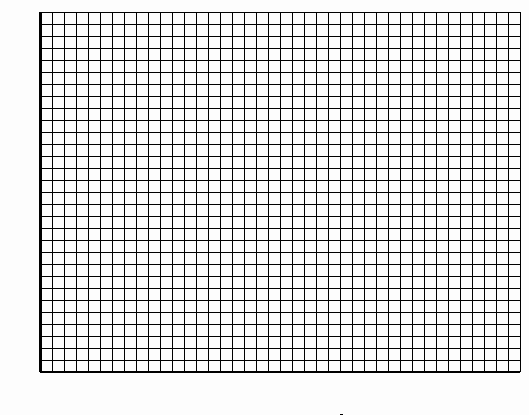PRINT THIS ANSWER SHEET TO FILL OUT AND TURN IN.

NAME_________________________________ CLASS____________________ DATE__________

### Beginner's Guide to Propulsion

Charles and Gay-Lussac's Law - Worksheet
1. Volume is directly proportional to the temperature means......

2. C =

3.  a. V=

b. V=

c. V=

d. V=

4. Plot these points on a graph, or use a graphing calculator. Describe the graph.5. How do the graphs compare?

6.  a. V =

b. V =

c. T =

d. T =

7. Were the answers the same? Why or why not?

8. Do you want to change your answer to question 1 or elaborate on it?